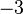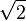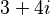# User:IssaRice/Computability and logic/Model as the representation and as that which is represented

From the preface (written by Jesús Mosterín) of María Manzano's Model Theory (p. ix):

In ordinary language, as well as in scientific language the word model is used in two distinct and opposite senses. They both have to do with the relation between a representation and that which is represented. The problem lies in that the model is sometimes identified with the first term of the relation (i.e. the representation), and sometimes with the second one (i.e. that which is represented). Thus, in the first sense, it is said that the artefact in reduced scale which represents a boat or an airplane is a model of the boat or the airplane. Also, in economy or in cosmology one speaks of econometric models or cosmological models, where those are understood as certain mathematical representations of the market or the universe. In mathematical logic, on the contrary, the representation is called theory, and that which is represented is a model of the theory. This use is contrary to the usual one in the empirical sciences, but it coincides with that of painters and photographers, when they speak of the model (that which is represented) as the object of the painting or the photograph (the representation). It is in this sense that one needs to understand the word model which appears in the theory of models developed by mathematical logicians and expounded in this book.
Example That which is represented The representation "Model" refers to
wikipedia:Scale model The actual physical object (e.g., boat or airplane) The copy/artifact The representation
wikipedia:Scientific model The physical world or reality (e.g., the economy in economics or the universe in cosmology) The mathematical description The representation
Art model Object of the art (e.g., the thing being painted or the thing being photographed) The artwork (e.g., the painting or the photograph) That which is represented
Model in mathematical logic The "mathematical reality" (e.g., arithmetic) Theory That which is represented

Remark 2.1.15 in Tao's Analysis I:

Historically, the realization that numbers could be treated axiomatically is very recent, not much more than a hundred years old. Before then, numbers were generally understood to be inextricably connected to some external concept, such as counting the cardinality of a set, measuring the length of a line segment, or the mass of a physical object, etc. This worked reasonably well, until one was forced to move from one number system to another; for instance, understanding numbers in terms of counting beads, for instance, is great for conceptualizing the numbers$3$ and$5$, but doesn’t work so well for$-3$ or$1/3$ or$\sqrt{2}$ or$3 + 4i$; thus each great advance in the theory of numbers – negative numbers, irrational numbers, complex numbers, even the number zero – led to a lot of unnecessary philosophical anguish. The great discovery of the late nineteenth century was that numbers can be understood abstractly via axioms, without necessarily needing a concrete model; of course a mathematician can use any of these models when it is convenient, to aid his or her intuition and understanding, but they can also be just as easily discarded when they begin to get in the way.

Tao's quote is a little tricky, because now we have three layers: (1) the physical objects that approximate number systems (e.g., counting beads); (2) the abstract mathematical structure (the number system); (3) the formal language theory (a bunch of symbols being shoved around on paper). And Tao seems to be using "model" to mean (1) rather than (2).

See also https://terrytao.wordpress.com/2015/09/29/275a-notes-0-foundations-of-probability-theory/ I think these notes are using "model" in yet another sense...